×

Search anything:

# Convolution Layer: The layer that takes over 70% of time in a Machine Learning model

#### Machine Learning (ML) convolutionGet this book -> Problems on Array: For Interviews and Competitive Programming

Convolutional Layer is the most important layer in a Machine Learning model where the important features from the input are extracted and where most of the computational time (>=70% of the total inference time) is spent.

Following this article, you will how a convolution layer works and the various concepts involved like: kernel size, feature map, padding, strides and others.

### Why is Convolution Layer Required?

One of the challenges in computer vision problems is that images can be very large and thus computationally expensive to operate upon. We need faster and computationally cheap algorithms for practical uses. A simple fully connected neural network won’t help.

For example, if an RGB image is of size 1000 X 1000 pixels, it will have 3 million features/inputs (3 million because each pixel has 3 parameters indicating the intensity of each of the 3 primary colours, named red, blue and green. So total features = 1000 X 1000 X 3 = 3 million) to the fully connected neural network.

If the hidden layer after the input layer contains 1000 activation units, then we will need a weight matrix of shape [1000, 3 million] which is 3 billion parameters only in the first layer and that’s extremely expensive computationally.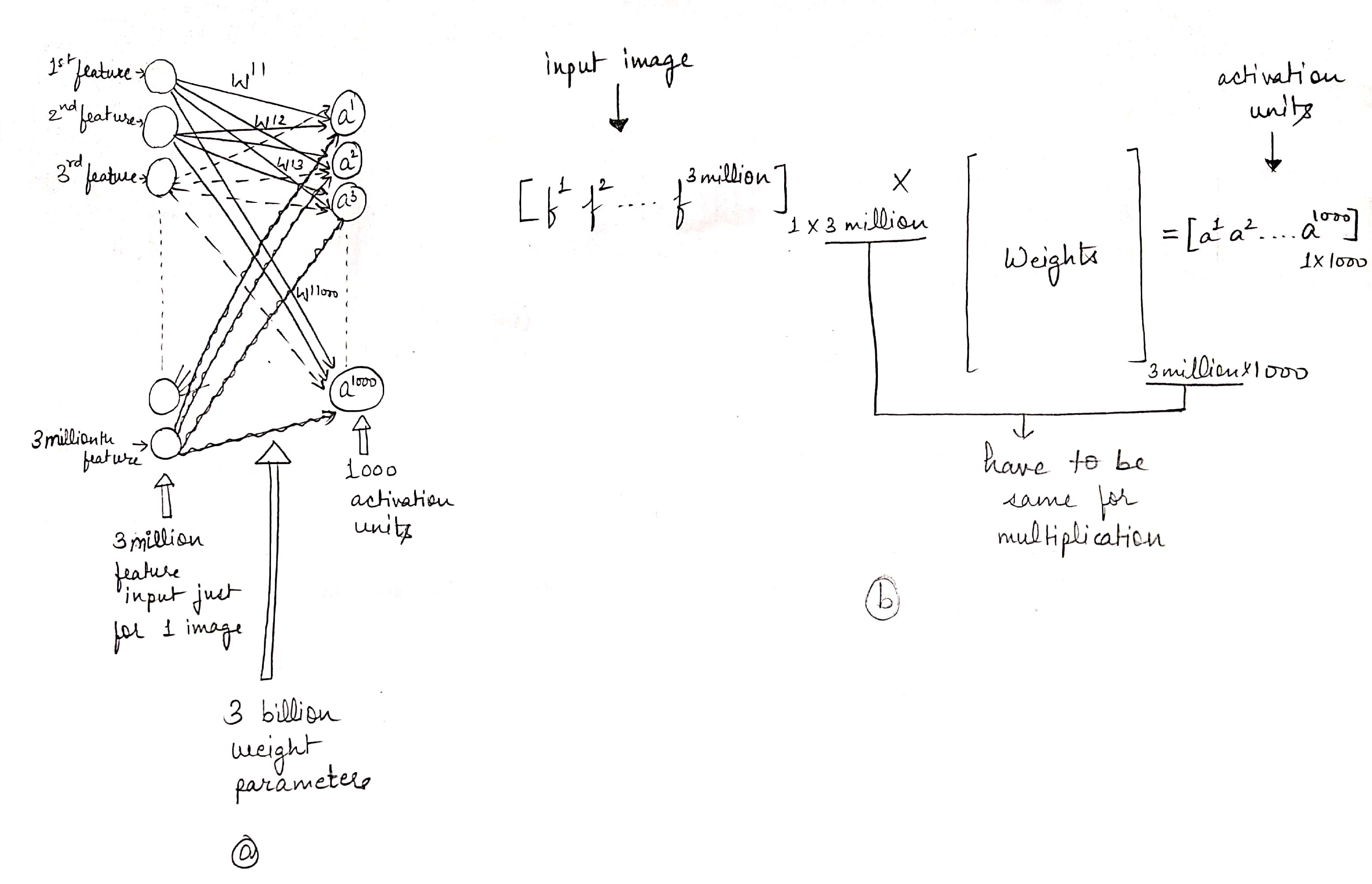This is where our beloved Convolution Layer comes to the rescue.

#### Following Convolution layer, which is the most computational expensive layer?

Fully connected layer
ReLU
Softmax
Pooling
Fully connected layer is the second most computational expensive layer

### Working of a Convolution Layer

The basic idea here is that instead of fully connecting all the inputs to all the output activation units in the next layer, we connect only a part of the inputs to the activation units. Here’s how: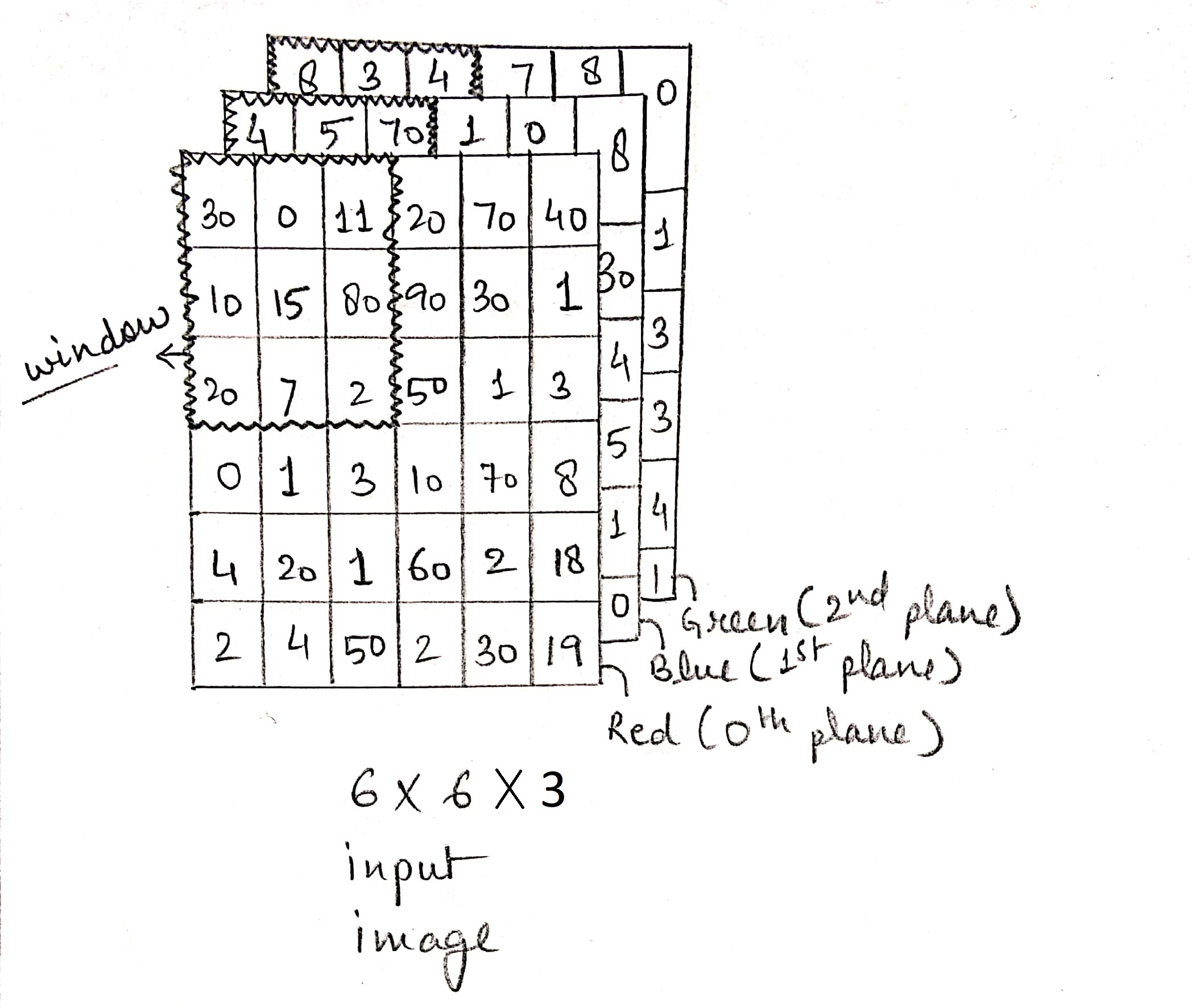The input image can be considered as a n X n X 3 matrix where each cell contains values ranging from 0 to 255 indicating the intensity of the colour (red, blue or green). Each of the 3 planes indicate the intensity of the colour red, blue and green respectively.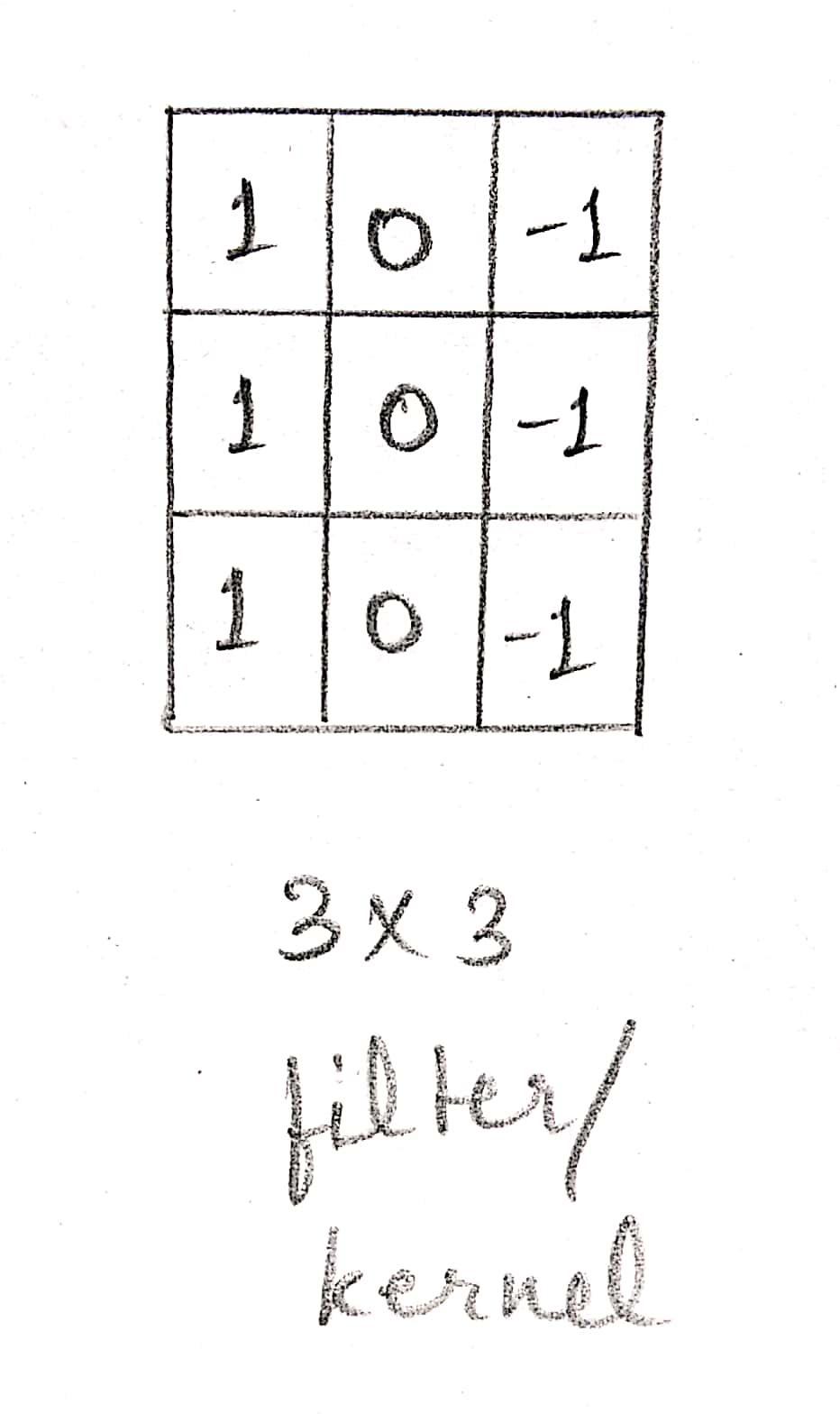#### Weight matrix / Kernel / Filter

The weight matrix, also known as filter or kernel, is an f X f matrix. Kernel size is the size of this kernel. Now, let's see how the convolution operation takes place.

Let us first take the 0-th plane (red plane) in the input matrix. Now we will have an f X f window sliding across this plane in specified steps/strides.

Initially the window is as shown in the figure below. We take the sub-matrix inside the window and perform element-wise matrix multiplication with the filter followed by the addition of the products of the elements as shown in the figure below.

Next we perform the same operation on the next two planes one by one ( Note that the input to a convolution layer can have any number of planes. So the operation will be performed on each plane ). Then we add the results from the three planes (If there were n number of planes in the input matrix then the we would have n results to be added up). The resultant of this final addition gives us the first element of output matrix.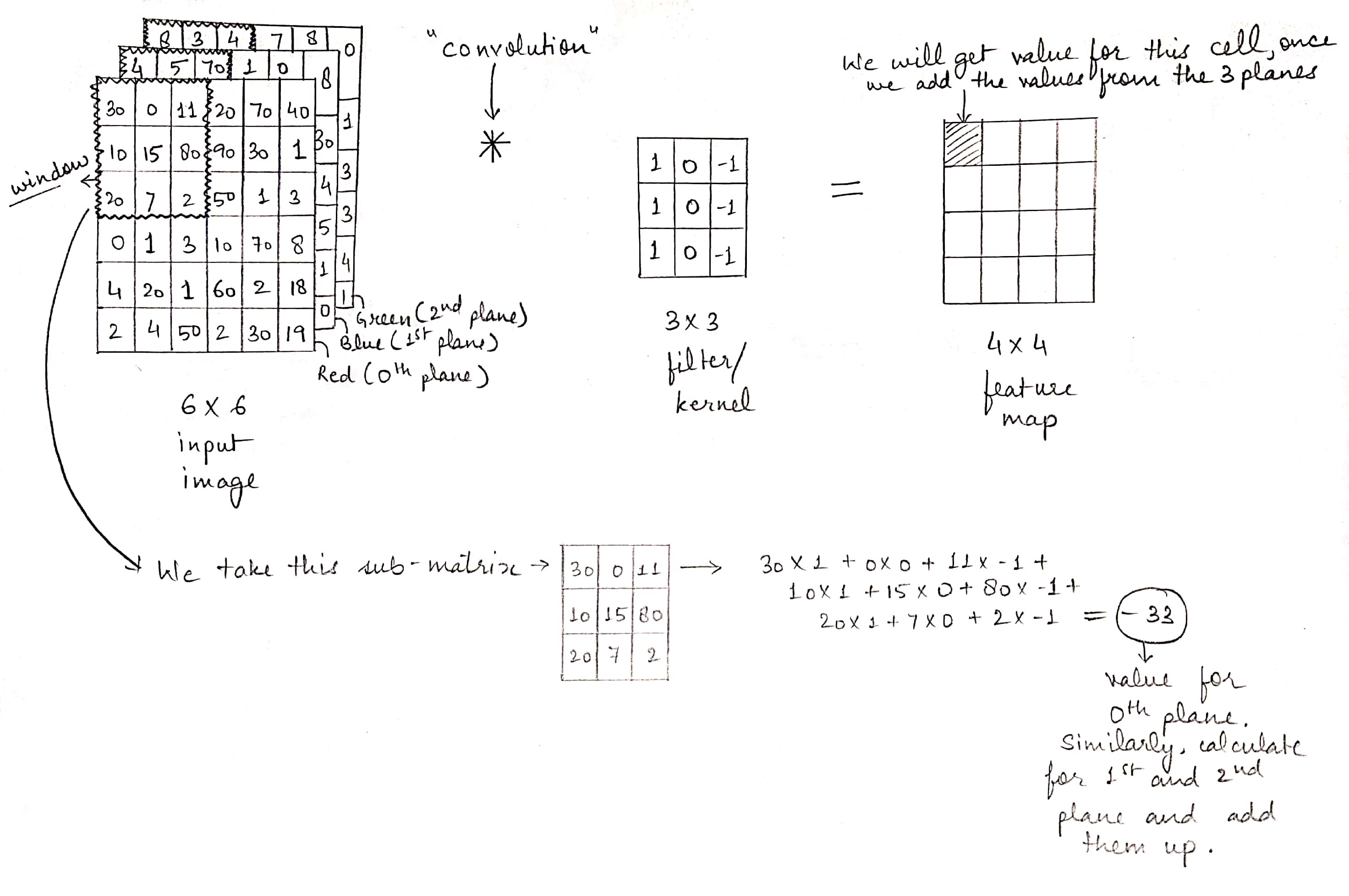#### Stride

Now we shift the window to the right by a specified number of steps called horizontal stride. If the stride, s = 1, the new position would be like this: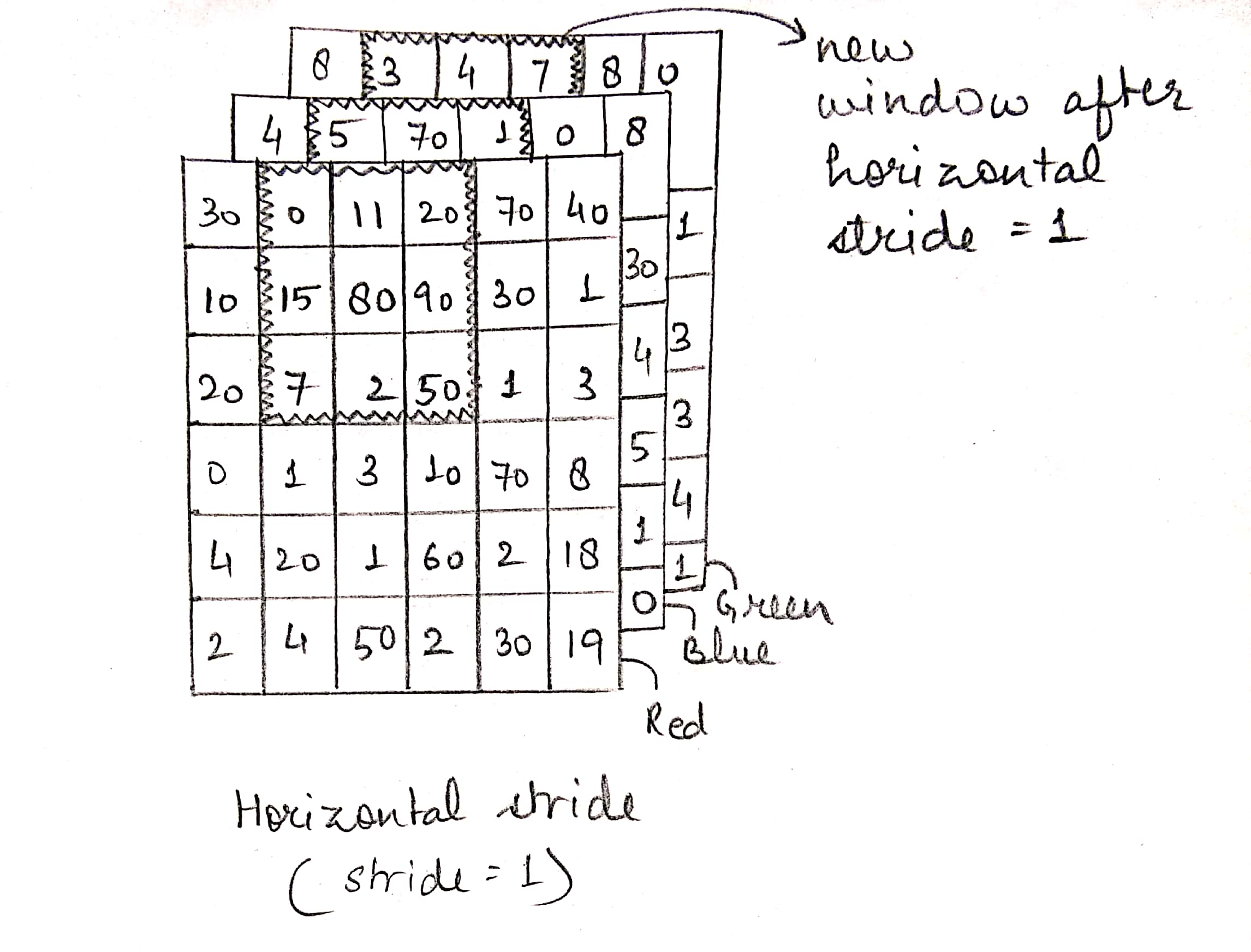We perform the same operation with the new sub-matrix and the filter to get the next element. We keep sliding the window until we reach the end horizontally, then we bring the window back to the left and shift it down by the vertical stride. If vertical stride is also 1, new position of the window will be like this: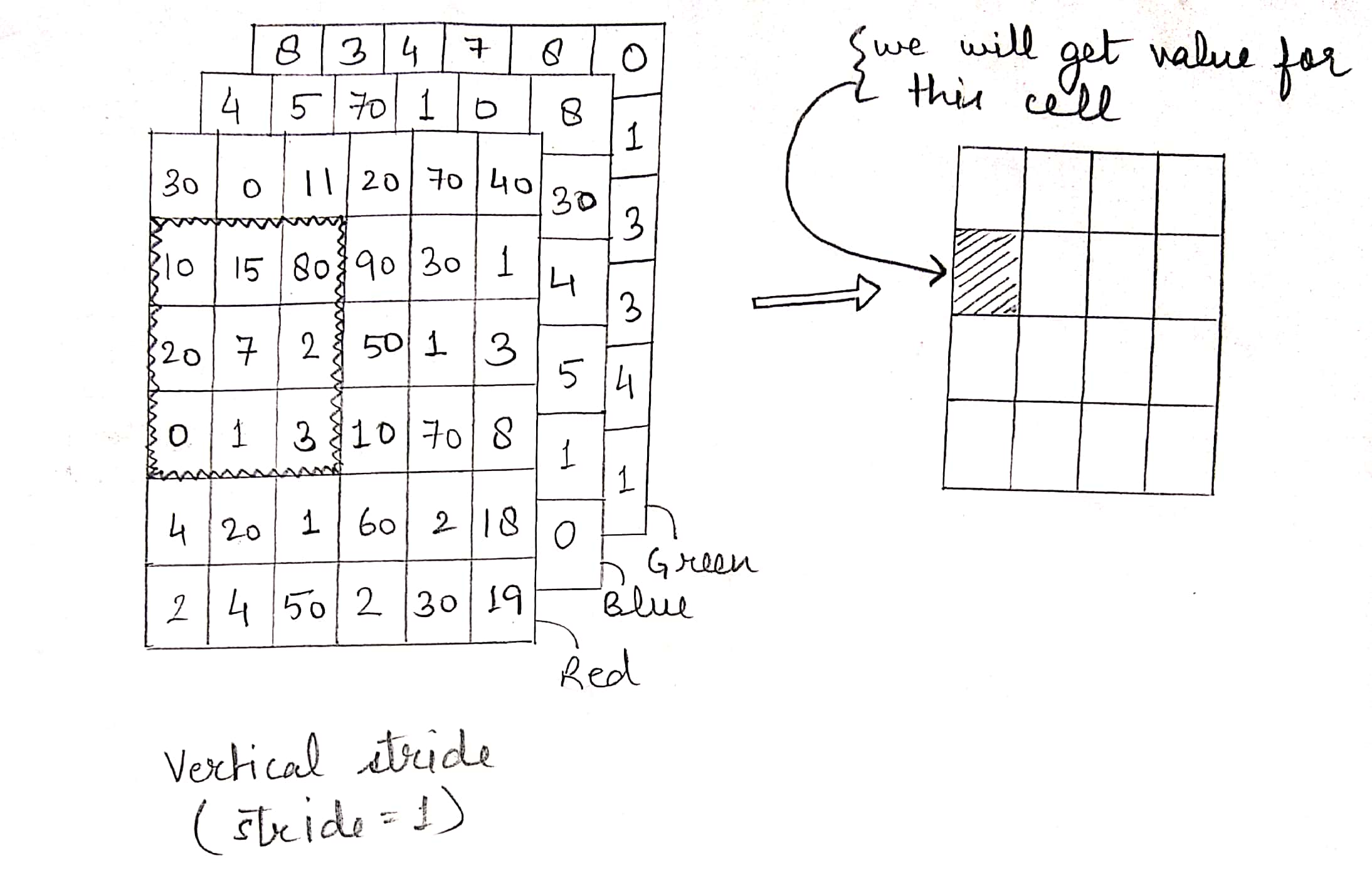#### Feature map

We repeat the process till we have covered all the elements of the input matrix. The resulting matrix is called the feature map.

Every feature map has its own bias term and this bias term is added to all the elements of the corresponding feature map. Once the bias terms are added, we get a new matrix. This new matrix is fed into the next layer (which is usually RelU layer) as an input matrix. Now we perform RelU activation function on each element of the feature map. The cells in the resultant matrix after we have applied RelU function, are nothing but activation units. Now as you can see that each activation unit is connected only to a particular portion of the input matrix unlike the conventional neural network where every activation unit is connected to the entire input. This was the whole idea.

We call the process CONVOLUTION and the input matrix is said to have convolved using the given filter. The layer where convolution takes place is called the Convolution layer.

As you can see, the number of parameters required have reduced by a significant amount making the process faster and computationally much less expensive.

Now let us suppose, we would like to preserve the information present in the pixels at the edge of the input matrix. Or we want the shape of the feature map to be the same as that of input matrix. We do so using padding. Padding is simply adding zeros on all sides of the input as shown in the figure. Now, if we perform the same convolution like we did before: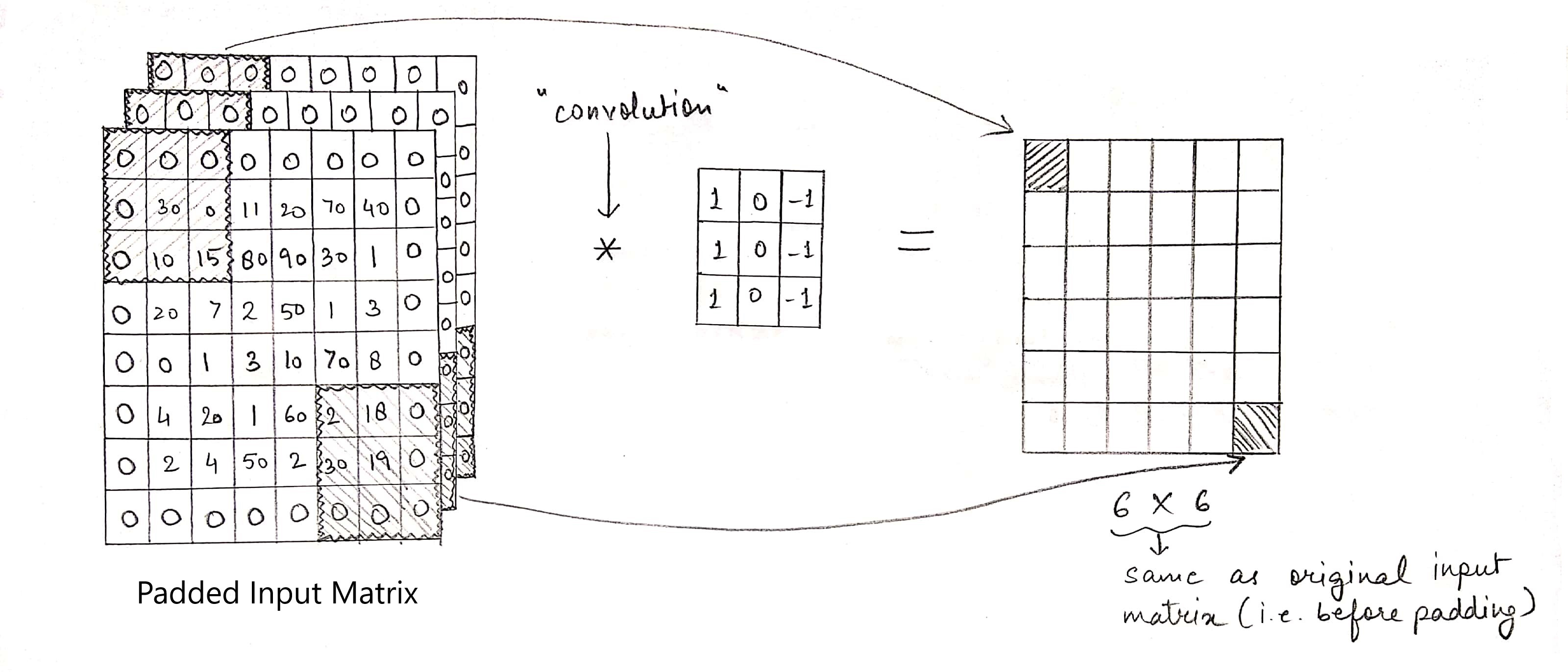### Shape calculation

The size of feature map is the same as input matrix. A formula can help here:
Let the filter shape be f X f; stride be s and padding be p. Let the input matrix be of shape n X n X z.

Then output matrix shape is : [((n+2p-f)/s)+1 , ((n+2p-f)/s)+1 , z]

#### What will be the shape of output matrix if filter shape is 3X3, stride is 2, padding is 2 and input image is of shape 256 X 256 X 3? in convolution layer

258 X 258 X 3
256 X 256 X 3
128 X 128 X 2
3 X 3 X 256

Note that here we can have several filters in a convolution layer each resulting in a particular feature map. So if there are 10 filters, we will have 10 feature maps. We stack these feature maps, one on top of another to get a new 3D matrix where each plane in this 3D matrix is a feature map.

If you make the convolution operation in TensorFlow you will find the function tf.nn.conv2d. In keras you will find Conv2d function.

### Example of working of a Convolution Layer

Enough with the theory. Let’s take an example to see the working.
Let suppose a filter detects a vertical edge. It would be like this:For the sake of simplicity, we’ll take a 2D grayscale image which has only one plane.
Convolution :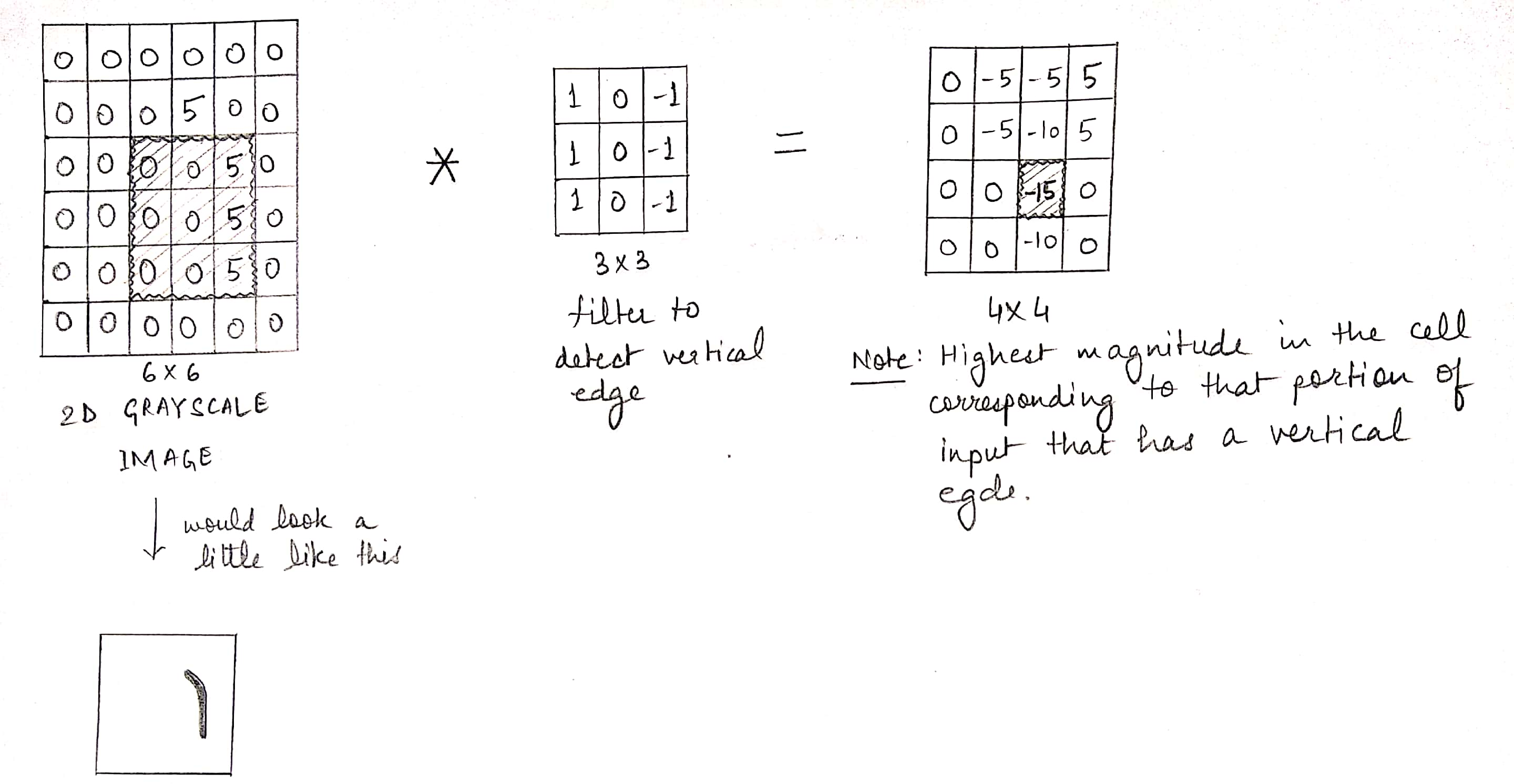As you can see, the part of the image where there was a vertical edge has higher value. Vertical edge is simply a feature in the image that was detected by our filter. We got a feature map for vertical edges. Similarly, we can detect horizontal or diagonal edges etc. For horizontal edge detection, we will have the following filter: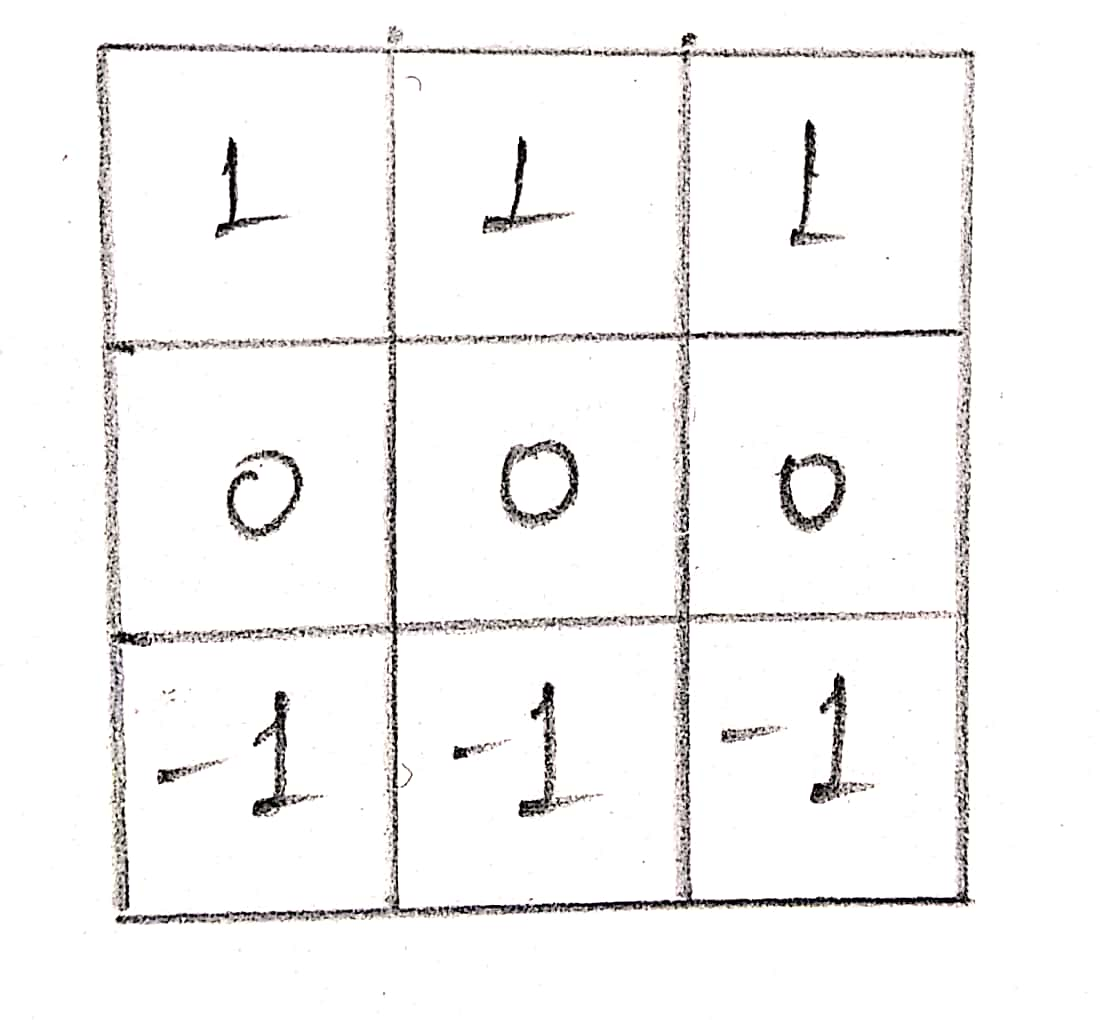In early convolution layers, these simple features are detected but as we go deeper into the Convolution Neural Network, more complex features are detected like faces, trees etc.

This was all about convolution layer. Thanks for reading. Hope you found it useful.